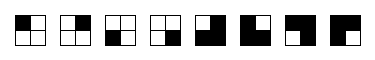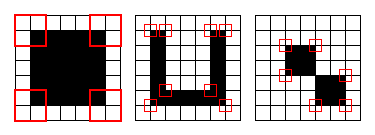### Problem Statement

Corners compression is a method of compressing black-and-white images to a list of so-called corners which works as follows.

The image to be compressed is a rectangular grid of pixels, where each pixel is either black or white. First the image is padded with a row of white pixels on the top and on the bottom and a column of white pixels on the left and on the right. All the following processing is done with the padded image.

Image rows are numbered from 0 (top row) to H-1 (bottom row), and image columns are numbered from 0 (left column) to W-1 (right column). Each pair of indices (i, j), 0 <= i < H-1, 0 <= j < W-1, defines a 2x2 block of pixels (i,j), (i+1,j), (i,j+1) and (i+1,j+1). To perform the compression, we consider all 2x2 blocks in the image. Blocks which contain an odd number of black pixels are classified as corners (the corresponding blocks are shown in the image below).The compression result is the list of corners - all pairs of indices (i, j) that define a 2x2 block which is a corner. The image below shows 3 separate images with all their corners marked.The set of corners resulting from this process, together with W and H (the dimensions of the padded image), uniquely identify the original image. To prove this, note that the image can be restored using the following process. Start with a HxW grid of pixels and set the color of the boundary pixels to white (these are the pixels of the padding rows and columns). If the rest of pixels are scanned, for example, in row-wise order (each row scanned from left to right), each pixel is the bottom-right pixel of a 2x2 block, with the other three pixels in this block being known (since they have been scanned earlier). Thus, the color of this pixel is uniquely defined by whether this block is a corner, since one color makes this block a corner, and the other doesn't.

You are given the list of corners in int[]s rows and cols. The coordinates of the i-th corner are (rows[i], cols[i]). Return the number of black pixels in the image which could have been compressed to this list of corners. If no finite image exists that could have been compressed to the given set of corners, return -1.

### Definition

 Class: CornersDecoding Method: blackPixels Parameters: int[], int[] Returns: long Method signature: long blackPixels(int[] rows, int[] cols) (be sure your method is public)

### Notes

-You are not given the dimensions of the original image (W and H), so the set of corners can be decoded to several images, but all these images will differ only due to extra white rows and columns on the bottom and right sides of the image, so they all will have the same number of black pixels.

### Constraints

-rows will contain between 0 and 50 elements, inclusive.
-cols will contain the same number of elements as rows.
-Each element of rows and cols will be between 0 and 100000, inclusive.
-All corners will be distinct.

### Examples

0)

 `{0, 0, 5, 5}` `{0, 5, 0, 5}`
`Returns: 25`
 This example represents the first image from the problem statement - a solid 5x5 square of black cells.
1)

 `{0, 0, 0, 0, 4, 4, 5, 5}` `{0, 1, 4, 5, 1, 4, 0, 5}`
`Returns: 13`
 This example represents the second image from the problem statement. Note that several corner blocks can overlap, i.e., have common pixels.
2)

 `{1, 1, 3, 3, 5, 5}` `{1, 3, 1, 5, 3, 5}`
`Returns: 8`
 This example represents the third image from the problem statement.
3)

 `{0, 0, 1, 2}` `{0, 2, 3, 4}`
`Returns: -1`
 No finite image could have been compressed to the given set of corners.

#### Problem url:

http://www.topcoder.com/stat?c=problem_statement&pm=10796

#### Problem stats url:

http://www.topcoder.com/tc?module=ProblemDetail&rd=14225&pm=10796

Nickolas

#### Testers:

PabloGilberto , ivan_metelsky , StevieT

#### Problem categories:

Encryption/Compression, Math